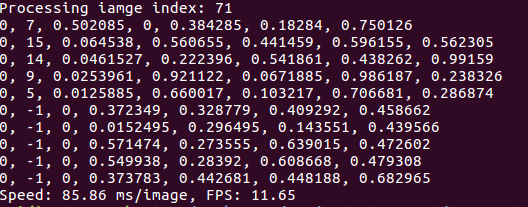# TensorRT&rsquo;s softmax plugin

Last week I implemented my ssd model using tensorRT, and I came cross some problems:

First, the result will change every time i run the inference, although the variation is slight. But I think it’s a unusual phenomenon:

the results are different between two detectons about image-71.

Second, when I deployed the code to Jetson, there was a big difference between the 1080ti and jetson tx2, see this:the confidence about image-71 is much lower, I don’t know why.

Last week I spent a lot of time looking for mistakes, and finally I solved the problem. The bug lies in the softmax plugin layer. But I have some question.

Firstly, I didn’t use the tensorrt’s built-in softmax layer and didn’t write the softmax plugin layer, the code I refered was:

``````__global__  void kernelSoftmax( float* x, int channels, float* y)
{
extern __shared__ float mem[];
__shared__ float sum_value;

float number = *(x + blockDim.x*blockIdx.x + threadIdx.x);
float number_exp = __expf(number);

y[blockDim.x*blockIdx.x + threadIdx.x] = __fdiv_rd(number_exp, sum_value);

}

void cudaSoftmax(int n, int channels,  float* x, float*y)
{
kernelSoftmax<<< (n/channels), channels, channels*sizeof(float)>>>( x, channels, y);
}
``````

I found it here: https://github.com/chenzhi1992/TensorRT-SSD/issues/11 and here: https://github.com/saikumarGadde/tensorrt-ssd-easy.

I didn’t know where the problem is until I print the softmax’s output vector:

: 0.220911, 0.000013, 0.000007, 0.000010, 0.000015, 0.000021, 0.000022, 0.000005, 0.000022, 0.000144, 0.000002, 0.000010, 0.000010, 0.000003, 0.000002, 0.000205, 0.000034, 0.000011, 0.000010, 0.000004, 0.000027,
: 0.142497, 0.000011, 0.000018, 0.000006, 0.000015, 0.000062, 0.000004, 0.000068, 0.000025, 0.000056, 0.000004, 0.000020, 0.000013, 0.000002, 0.000002, 0.000189, 0.000061, 0.000020, 0.000007, 0.000004, 0.000030,

the sum of the bouding box’s was not equal to 1， that’s the problem. Actually, many layers’ softmax output vector were abnormal, I just put these two as a demonstration. So I rewrote the softmax plugin layer accordding to the caffe’s softmax layer, and now the softmax is working properly.

But I don’t know why the code above can’t work properly. Firstly I thought it was the overflow problem of softmax, I printed the layer’s output before the softmax about these two bounding box.

: 9.448281, -0.305943, -0.866351, -0.567637, -0.179759, 0.209678, 0.253052, -1.152503, 0.246780, 2.111533, -2.058532, -0.569763, -0.556518, -1.891119, -2.310432, 2.466331, 0.668827, -0.453421, -0.569193, -1.553695, 0.450949,
: 8.824076, -0.685308, -0.170986, -1.321945, -0.327469, 1.080537, -1.752859, 1.176885, 0.182785, 0.987870, -1.665360, -0.023708, -0.513034, -2.233975, -2.505888, 2.199900, 1.067560, -0.024934, -1.046106, -1.624071, 0.364584,

It doesn’t seem to be this problem. I put these vector to python numpy and computed the softmax vector, the result was normal. I have tested this at float32 and float64 and different versions of softmax(consider the overflow or not).

It looks like the kernel function is not synchronized? But there is a __syncthreads(）statement. so where is the problem about this kernel function? I am not familiar with the cuda kernel function.